When working with data in Pandas, it is sometimes necessary to merge and concatenate multiple data, the most common of which include merging multiple split files.

 `````` 1 2 3 4 5 6 7 8 9 10 11 12 13 14 15 16 17 `````` ``````import pandas as pd import glob file_list = glob.glob('data/*') df_list = [] for file in file_list: df_temp = pd.read_csv(file, sep="
import pandas as pd import glob file_list = glob.glob('data/*') df_list = [] for file in file_list: df_temp = pd.read_csv(file, sep="\001", header=None, na_values=['\\N']) df_list.append(df_temp) df = pd.concat(df_list, ignore_index=True)
1", header=None, na_values=['\\N']) df_list.append(df_temp) df = pd.concat(df_list, ignore_index=True) ``````

There are a total of four methods for merging data in Pandas.

• append()
• concat()
• merge() or df.merge()
• join()

## df.append()

df.append () is better understood, is the original data in the append data, the syntax: df1.append (df2, sort=False), the effect is similar to UNION ALL in SQL.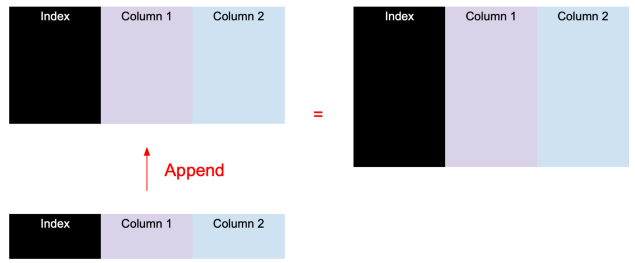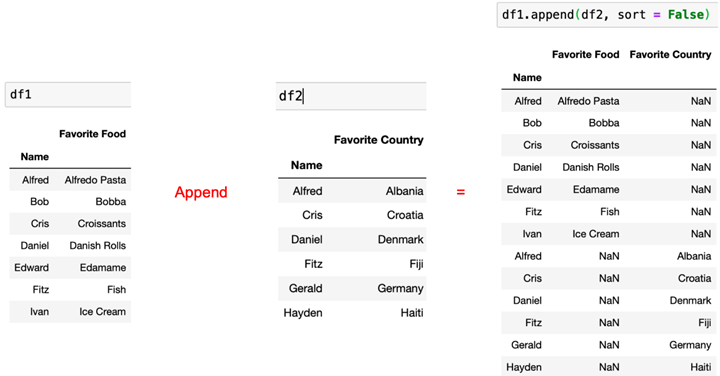## pd.concat()

pd.concat() is equivalent to the enhanced version of df.append(). It supports merging multiple DataFrames in addition to vertical (portrait) merging, but also horizontal (landscape) merging.

Vertical (vertical) merge: pd.concat([df1, df2], sort = False)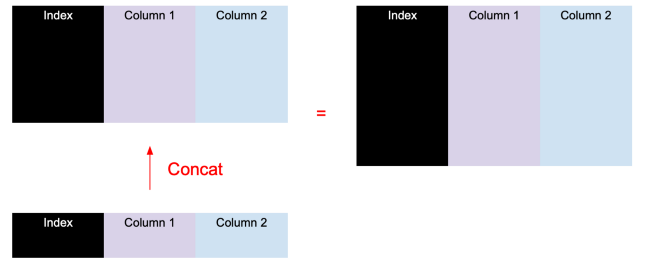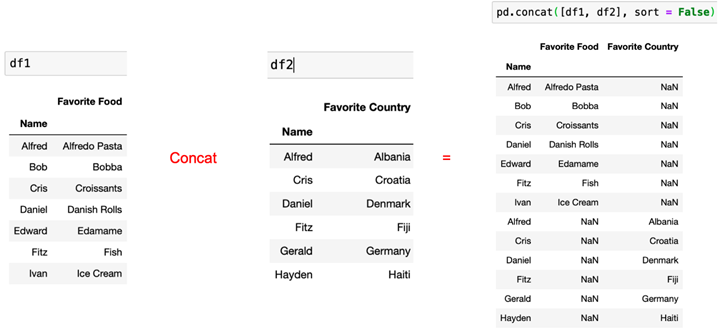Horizontal (horizontal) merge: pd.concat([df1, df2], axis = 1, sort = False)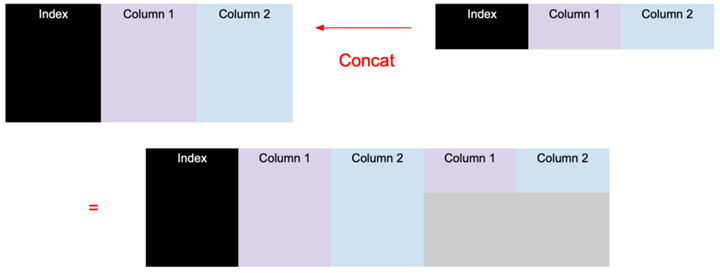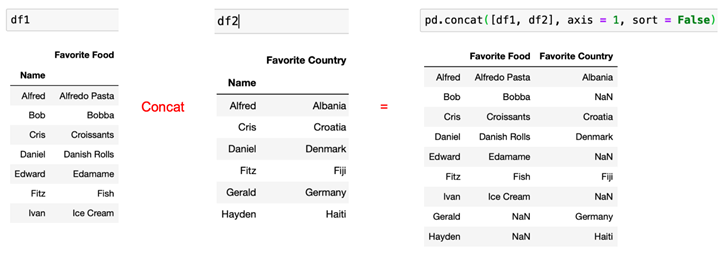pandas.concat(objs, axis=0, join=‘outer’, ignore_index=False, keys=None, levels=None, names=None, verify_ integrity=False, sort=False, copy=True)

Parameter description.

• objs: The sequence or mapping of Pandas Series or DataFrame objects to join.
• join: join method (inner or outer)
• axis: join along a row (axis=0) or column (axis=1)
• ignore_index: if True, the index of the original DataFrames is ignored.
• keys: order of adding identifiers to the result index
• levels: the levels used to create the MultiIndex
• names: the names of the levels in the MultiIndex
• verify_integrity: boolean. If True, check for duplicates.
• sort: boolean. When join is outer, sort the non-concatenation axes if they are not already aligned.
• copy: Boolean. If False, avoid unnecessary data copying.

## pd.merge() or df.merge()

pandas provides a method similar to the join operation of relational databases merage, which can join rows in different DataFrames based on one or more keys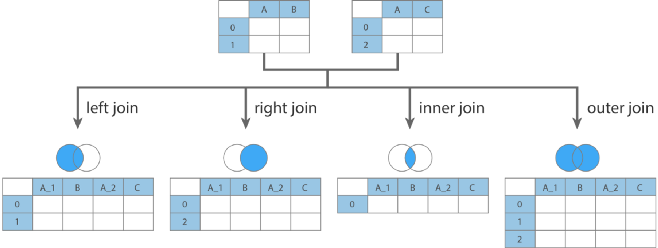DataFrame.merge(right, how=’inner’, on=None, left_on=None, right_on=None, left_index=False, right_index=False, sort=False, suffixes=(‘_x’, ‘_y’), copy=True, indicator=False, validate=None)

Parameter Description.

• right: the DataFrame on the right side when connected
• how: join method, optional values {’left’, ‘right’, ‘outer’, ‘inner’, ‘cross’}, default ‘inner’
• left: similar to SQL’s left join
• right: similar to SQL’s right join
• outer: SQL-like full join
• inner: SQL-like inner join
• cross: SQL-like cross join (Cartesian product)
• on: similar to SQL’s on, requires both left and right DataFrame to have the same column name.
• left_on: the name of the column associated with the left Dataframe
• right_on: the name of the column associated with the right Dataframe, which can be different from the name of the left one.
• left_index: use the row index in the left DataFrame as the join key.
• right_index: use the row index in the right DataFrame as the concatenation key.
• sort: default is True to sort the merged data, set to False to improve performance.
• suffixes: a tuple of string values, used to specify the suffix name to be appended to the column name when the same column name exists in the left and right DataFrame, default is (’_x’, ‘_y’).
• copy: default is True, always copy data into the data structure, set to False to improve performance.
• indicator: shows the source of the data in the merged data
• validate: validation option
• “one_to_one” or “1:1”: determines if the left table is one-to-one with the right table
• “one_to_many” or “1:m”: Determines if the left table is one-to-many with the right table
• “many_to_one” or “m:1”: Determines if the left table is many-to-one with the right table
• “many_to_many” or “m:m”: determines if the left table and the right table are many-to-many

## df.join()

df.join() is the built-in join method of dataframe, with index as the aligned column by default. The function is weaker than merge(), so we won’t introduce it in detail here.

DataFrame.join(other, on=None, how=‘left’, lsuffix=", rsuffix=", sort=False)

Parameter description.

• other: the right DataFrame
• on: same as above
• how: same as above
• lsuffix: the suffix of the duplicate column in the left DataFrame
• rsuffix: the suffix of the repeating column in the right DataFrame
• sort: same as above